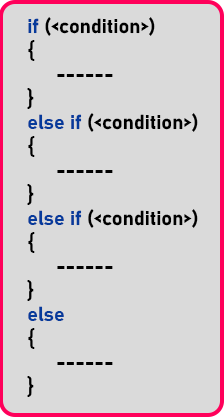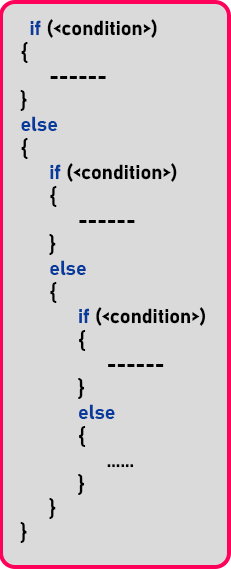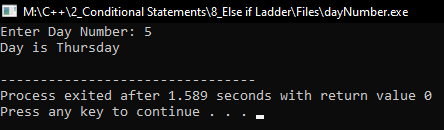# Else If Ladder in C++

## Else If Ladder in C++ with Examples:

In this article, I am going to discuss Else If Ladder in C++ with Examples. Please read our previous article where we discussed How to Display Grades for Student Marks in C++ with Examples. When we have multiple conditions to evaluate then we go for Ladder–if statements.

##### Else If Ladder in C++:

The extension of ‘nested if’ is known as else – if ladder. If we have more than one ‘nested if’ then it can be easily framed like else if ladder. This is very easy for writing otherwise too many ‘nested if’ will create confusion for a programmer and even the code will not be easily readable. So let us see how we can frame the ‘else if’ ladder for ‘nested if’.This is the ‘if-else’ ladder. 1st is if condition and if condition will true then if block will be executed. 2nd is the if-else condition, if the 1st ‘if’ condition fails then this ‘if-else’ block will be executed. Note – Instead of writing this condition nested, we have written that condition in ‘if-else’. 3rd is again the if-else condition, if the previous ‘if else’ condition fails then this ‘if-else’ block, will be executed. 4th is the else part, if all the previous condition fails then this else block will be executed. Now below is the nested form of the above ‘if-else’ ladder:If we write ‘nested if’ then it looks very congested. So, it’s better to write an if-else ladder instead of the ‘nested if’. Now let us write a program using the ‘if-else’ ladder. The program is we will take a day number and we will display day name will, the name means ‘1’ is Sunday, ‘2’ is Monday, ‘3’ is Tuesday and so on.

##### Program to Print Day Names using Else If Ladder in C++:
```#include <iostream>
using namespace std;

int main()
{
int day;

cout << "Enter Day Number: ";
cin >> day;
cout << "Day is ";

if (day == 1)
cout << "Sunday" << endl;
else if (day == 2)
cout << "Monday" << endl;
else if (day == 3)
cout << "Tuesday" << endl;
else if (day == 4)
cout << "Wednesday" << endl;
else if (day == 5)
cout << "Thursday" << endl;
else if (day == 6)
cout << "Friday" << endl;
else
cout << "Saturday" << endl;

return 0;
}
```
###### Output:In the next article, I am going to discuss Short Circuit in C++ with Examples. Here, in this article, I try to explain Else If Ladder in C++ with Examples, and I hope you enjoy this Else If Ladder in C++ with Examples article.